Elite indicators :) - page 548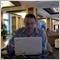Moderator
112873

So, good settings for this EA may be difficult to find. But from what I foind for now: Ichimoku cloud may be on M15 timeframe when EA is trading on M1 timeframe. So, we will have MTF EA anyway.

I think - this MTF settings may work: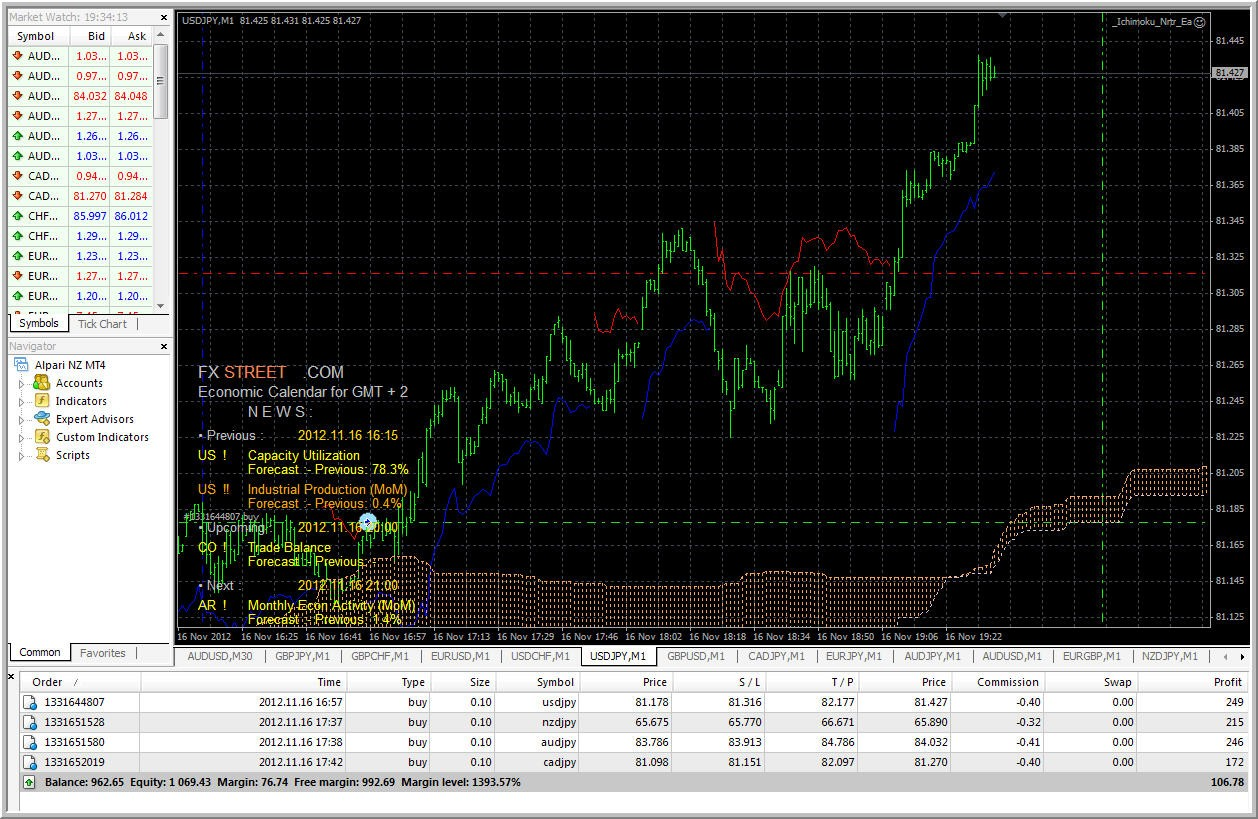I will test it a little more and I think - this EA may be uploaded after that.

======

I am writing it for myself just to find the posts about where it was started for example:

This post is continuation of the post 5395, post 5396, post 5402, post 5404, post 5413, post 5423, post 5425, post 5427, post 5431 and post 5472. This first version is on post 5491.

Files:531

Is there any chance you could add a trend buffer to this indicator??

Thank you

Ray

Files:148

Tma+cg

This is a great looking indicator.

However, sometimes a new arrow will appear on a history candle.

At other times, an existing arrow on a history candle will disappear.

Can this behavior be fixed??

Thank you.

Files:
tmacg.mq4 10 kb9496

michaelB:
This is a great looking indicator.

However, sometimes a new arrow will appear on a history candle.

At other times, an existing arrow on a history candle will disappear.

Can this behavior be fixed??

Thank you.

MichaelB, only thing i could find is for the arrows when there was a signal for the price leaving the lower band instead of using that price low it was using the price high, all i can figure about the disappearing is the band itself would cover the arrow, anyway might try this one and see if it is right.

Files:
tmacg-1.mq4 10 kbModerator
112873

This is some trades of this EA (very first version) with MTF settings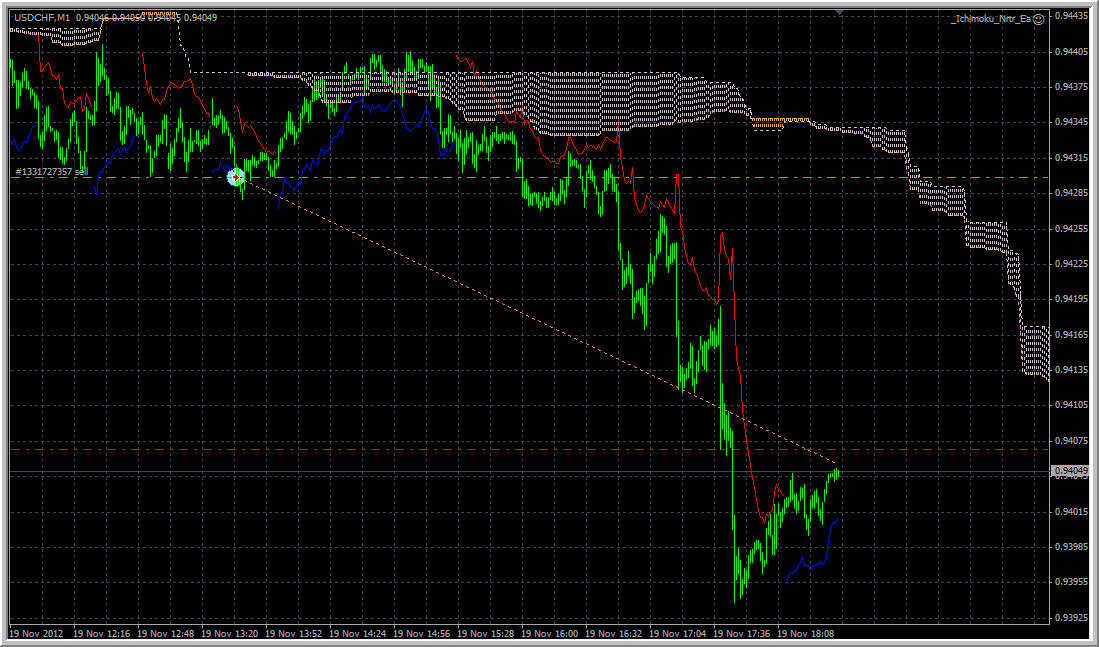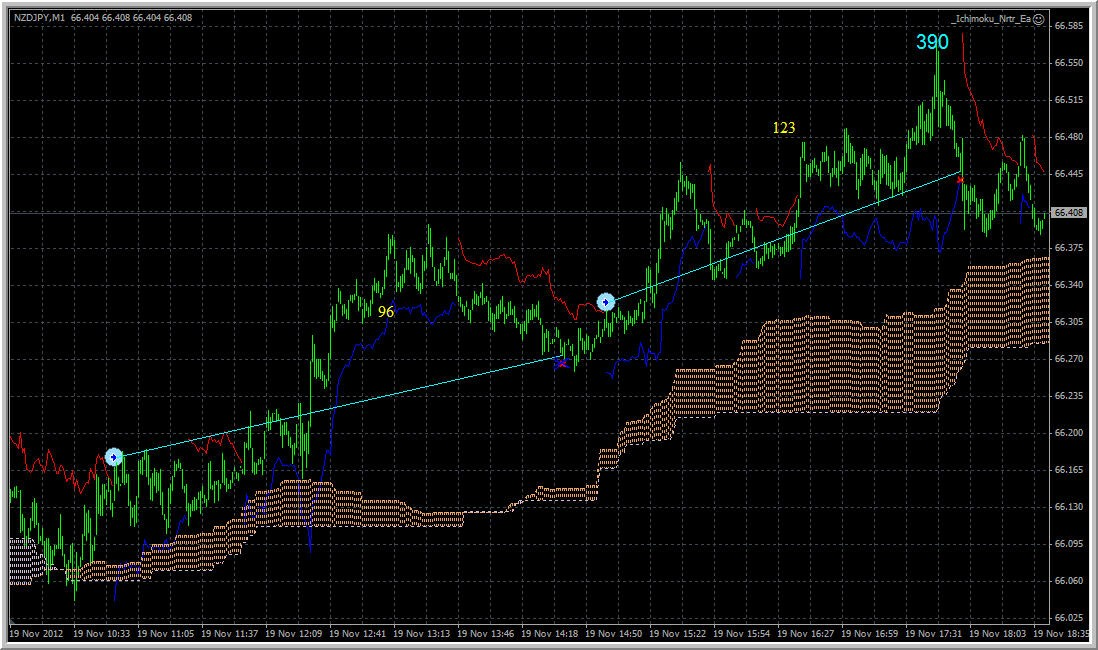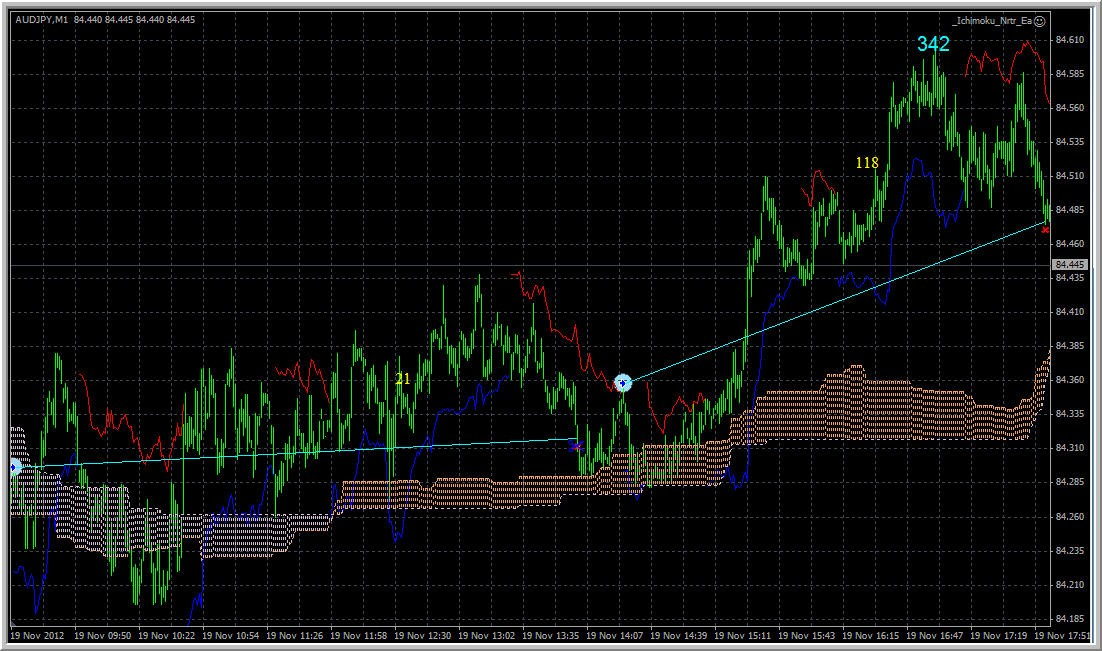======

I am writing it for myself just to find the posts about where it was started for example:

This post is continuation of the post 5395, post 5396, post 5402, post 5404, post 5413, post 5423, post 5425, post 5427, post 5431 and post 5472. This first version is on post 5491. This first version is on post 5491.Moderator
112873

This is updated situation with this EA (very very first version) with MTF settings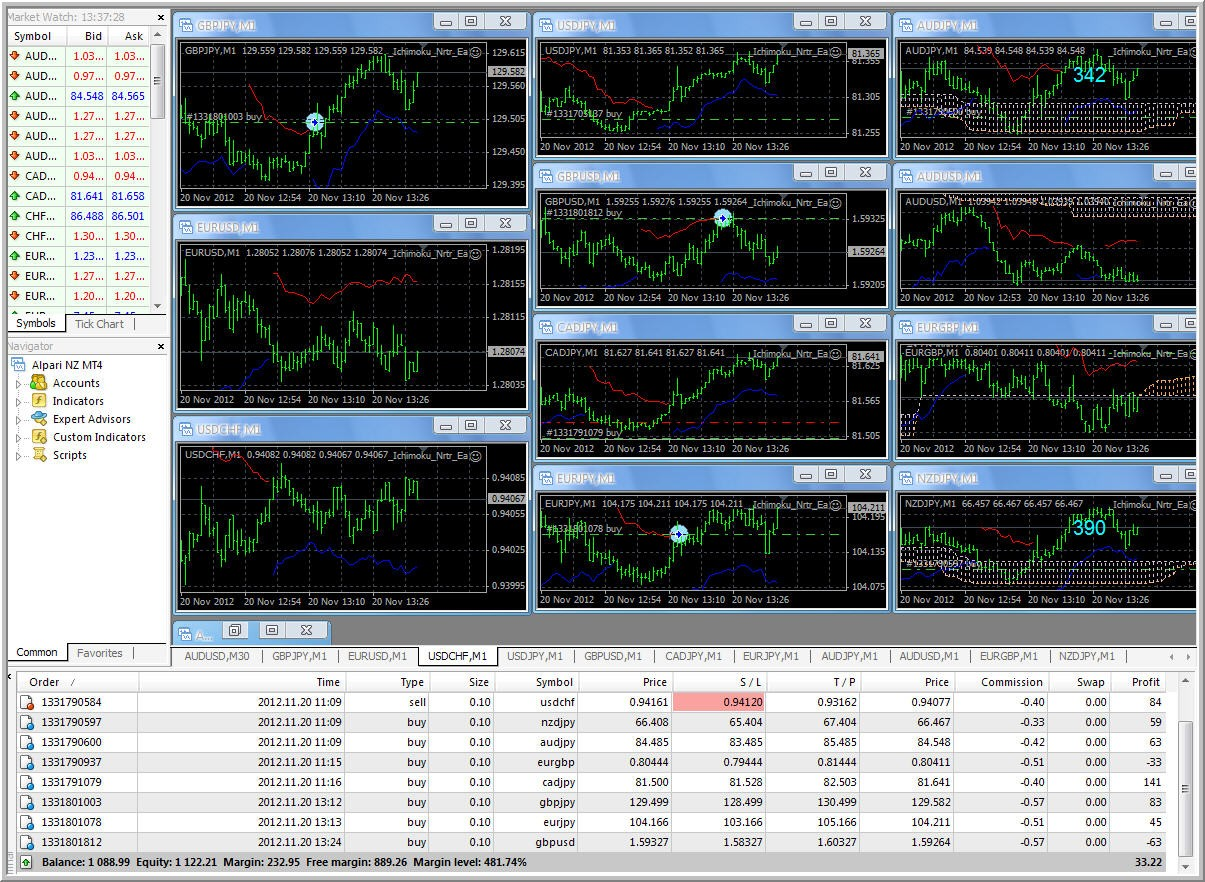Seems profitable (we can compare the im ageform soem perevious posts with this one - same account).

I think - we can upload this EA ... in the end of this week for example ...

But please note - it is very first version.

I am trading it using home pc and on the following way: swithing pc on in the morning, EA is trading, switching off in the evening.

======

I am writing it for myself just to find the posts about where it was started for example:

This post is continuation of the post 5395, post 5396, post 5402, post 5404, post 5413, post 5423, post 5425, post 5427, post 5431 and post 5472. This first version is on post 5491.

Files:247

RSX_MA_FullSSA with its inverse on same indicator

altoronto,

Changeable symbol added (so now you can chose the symbol for which to display value). Since from the experience I know that this way is used sometimes for correlations monitoring, added the invert option too (so you can invert the value around the 0 axis) That way I think all the angles are covered. Here is an example of a "foreign" symbol used with inverted values for the upper instance of the indicator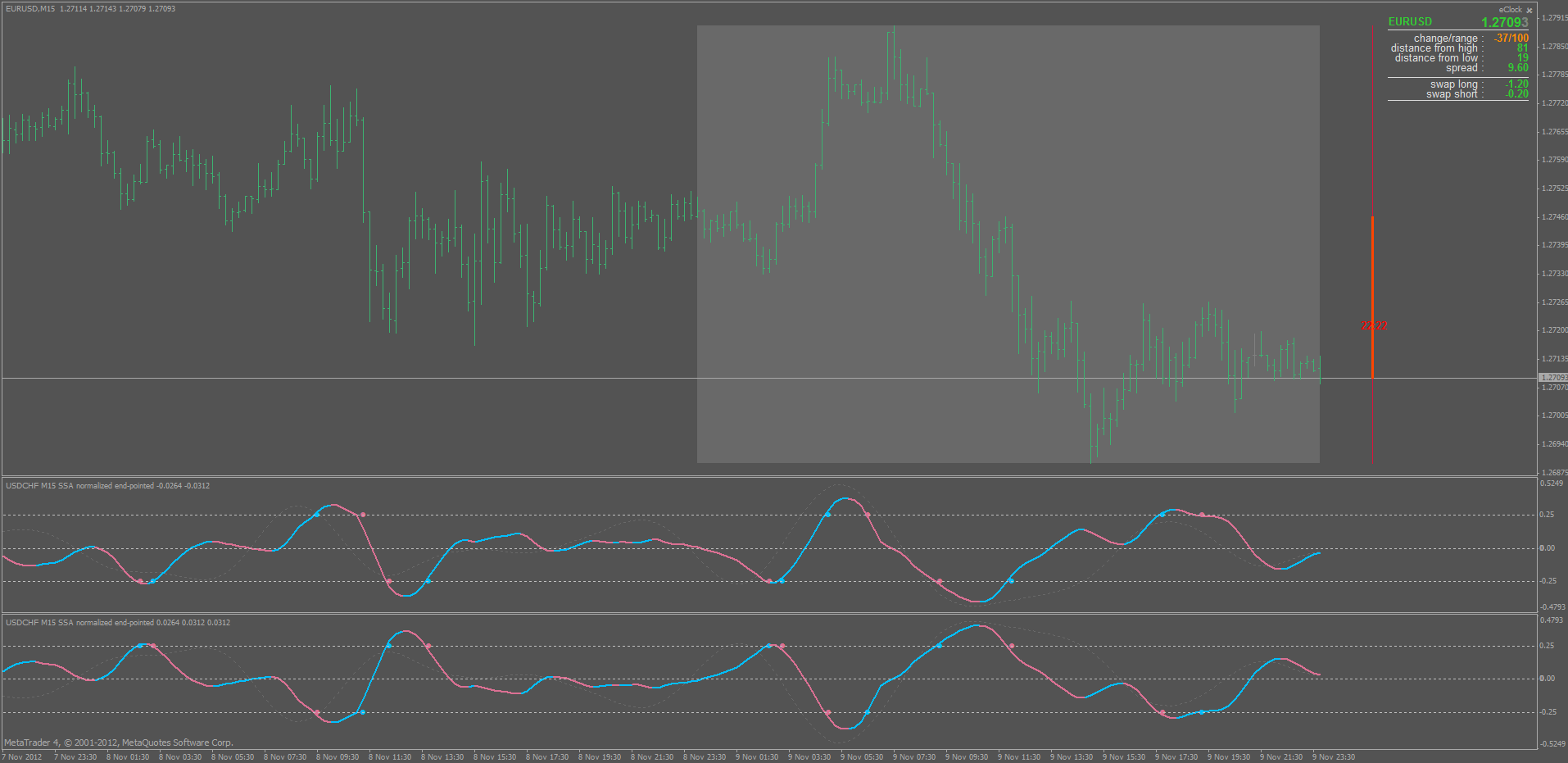I'm very new to this wonderful website and I'm wondering if you could make a variation to the SSAs indicators I've seen here. I got a high intensity CPU indicator from a diff site that I think is really great except it eventually crashes my computer. It is called RSX_MA_FullSSAaa (by a fellow named ESSAM) and it draws 3 diff color lines [wider to narrower] above the 0 line its inverse companion indicator is 3 diff color lines overlaid on the same indicator window area below the 0 line. if it is possible to make it so that it uses less CPU, that would be terrific.

the inputs are:

length 14

count bars 300

Ma type 1

Ma Period 8

Lag 20

Num Comps 2

PeriodNom 20

N 300

Unfortunately I don't know how to upload a picture of my metatrader chart to this screen. I tried to copy and paste the indicators to this space but that doesn't work either. any help and advice is really greatly appreciated. thank you very much in advance for all your efforts here.

David247

more info on RSX_MA_FullSSA with its inverse on same indicator

I copied picture and indicators and template from original free source

I'm very new to this wonderful website and I'm wondering if you could make a variation to the SSAs indicators I've seen here. I got a high intensity CPU indicator from a diff site that I think is really great except it eventually crashes my computer. It is called RSX_MA_FullSSAaa (by a fellow named ESSAM) and it draws 3 diff color lines [wider to narrower] above the 0 line its inverse companion indicator is 3 diff color lines overlaid on the same indicator window area below the 0 line. if it is possible to make it so that it uses less CPU, that would be terrific.

the inputs are:

length 14

count bars 300

Ma type 1

Ma Period 8

Lag 20

Num Comps 2

PeriodNom 20

N 300

Unfortunately I don't know how to upload a picture of my metatrader chart to this screen. I tried to copy and paste the indicators to this space but that doesn't work either. any help and advice is really greatly appreciated. thank you very much in advance for all your efforts here.

David247

info on template on RSX_Ma_FullSSAaa indicator

try once again

symbol=EURUSD

period=60

leftpos=1921

digits=5

scale=4

graph=0

fore=0

grid=0

volume=0

scroll=1

shift=1

ohlc=1

days=0

descriptions=0

shift_size=20

fixed_pos=0

window_left=66

window_top=87

window_right=876

window_bottom=572

window_type=3

background_color=9470064

foreground_color=0

barup_color=65280

bardown_color=65280

bullcandle_color=0

bearcandle_color=16777215

chartline_color=65280

volumes_color=3329330

grid_color=10061943

stops_color=255

height=100

name=main

height=50

name=Custom Indicator

name=RSX_MA_FullSSAaa200000\$

flags=347

window_num=1

Lengh=14

CountBars=300

MaType=1

MaPeriod=8

Lag=20

NumComps=2

PeriodNorm=20

N=300

shift_0=0

draw_0=0

color_0=0

style_0=0

weight_0=1

shift_1=0

draw_1=0

color_1=255

style_1=0

weight_1=1

shift_2=0

draw_2=0

color_2=65535

style_2=0

weight_2=0

min=-0.900000

max=0.900000

period_flags=0

show_data=1

name=Custom Indicator

name=RSX_MA_FullSSAaa200000\$\$

flags=347

window_num=1

Lengh=14

CountBars=300

MaType=1

MaPeriod=8

Lag=20

NumComps=2

PeriodNorm=20

N=300

shift_0=0

draw_0=0

color_0=16777215

style_0=0

weight_0=1

shift_1=0

draw_1=0

color_1=16711680

style_1=0

weight_1=1

shift_2=0

draw_2=0

color_2=65280

style_2=0

weight_2=0

min=-0.900000

max=0.900000

period_flags=0

show_data=1

I copied picture and indicators and template from original free source247

picture finally i hope

I hope this picture finally downloads- I"m very sorry my ignorance created so much redundancy.

David

try once again

symbol=EURUSD

period=60

leftpos=1921

digits=5

scale=4

graph=0

fore=0

grid=0

volume=0

scroll=1

shift=1

ohlc=1

days=0

descriptions=0

shift_size=20

fixed_pos=0

window_left=66

window_top=87

window_right=876

window_bottom=572

window_type=3

background_color=9470064

foreground_color=0

barup_color=65280

bardown_color=65280

bullcandle_color=0

bearcandle_color=16777215

chartline_color=65280

volumes_color=3329330

grid_color=10061943

stops_color=255

height=100

name=main

height=50

name=Custom Indicator

name=RSX_MA_FullSSAaa200000\$

flags=347

window_num=1

Lengh=14

CountBars=300

MaType=1

MaPeriod=8

Lag=20

NumComps=2

PeriodNorm=20

N=300

shift_0=0

draw_0=0

color_0=0

style_0=0

weight_0=1

shift_1=0

draw_1=0

color_1=255

style_1=0

weight_1=1

shift_2=0

draw_2=0

color_2=65535

style_2=0

weight_2=0

min=-0.900000

max=0.900000

period_flags=0

show_data=1

name=Custom Indicator

name=RSX_MA_FullSSAaa200000\$\$

flags=347

window_num=1

Lengh=14

CountBars=300

MaType=1

MaPeriod=8

Lag=20

NumComps=2

PeriodNorm=20

N=300

shift_0=0

draw_0=0

color_0=16777215

style_0=0

weight_0=1

shift_1=0

draw_1=0

color_1=16711680

style_1=0

weight_1=1

shift_2=0

draw_2=0

color_2=65280

style_2=0

weight_2=0

min=-0.900000

max=0.900000

period_flags=0

show_data=1